Taylor series operator is linear

This article gives a statement of the form that a certain operator from a space of functions to another space of functions is a linear operator, i.e., applying the operator to the sum of two functions gives the sum of the applications to each function, and applying it to a scalar multiple of a function gives the same scalar multiple of its application to the function.

Statement

1. Additivity: Suppose$f$ and$g$ are functions defined on subsets of reals that are both infinitely differentiable functions at a point$x_0$ which is in the domain of both functions. Then,$f + g$ is infinitely differentiable at$x_0$ and the Taylor series of$f + g$ about$x_0$ is the sum of the Taylor series of$f$ at$x_0$ and the Taylor series of$g$ at$x_0$. Note that we add Taylor series formally by adding the coefficients for each power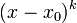$(x - x_0)^k$.
2. Scalar multiples: Suppose$f$ is a function defined on a subset of the reals and it is infinitely differentiable at a point$x_0$ in its domain. Suppose$\lambda$ is a real number. Then,$\lambda f$ is infinitely differentiable at$x_0$ and the Taylor series of the function$\lambda f$ at$x_0$ is$\lambda$ times the Taylor series of$f$ at$x_0$.

Facts used

1. Differentiation is linear, repeated differentiation is linear

Proof

Given:$f$ and$g$ are functions defined on subsets of reals that are both infinitely differentiable functions at a point$x_0$ which is in the domain of both functions.

To prove:$f + g$ is infinitely differentiable at$x_0$ and the Taylor series of$f + g$ about$x_0$ is the sum of the Taylor series of$f$ at$x_0$ and the Taylor series of$g$ at$x_0$.

Proof: By Fact (1), we have: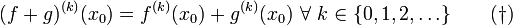$(f + g)^{(k)}(x_0) = f^{(k)}(x_0) + g^{(k)}(x_0)\ \forall \ k \in \{ 0,1,2,\dots \} \qquad (\dagger)$

with equality holding whenever the right side makes sense. Since by assumption, the right side always makes sense, the left side always makes sense, so$f + g$ is infinitely differentiable at$x_0$ and it makes sense to take its Taylor series.

We recall the expressions for the Taylor series: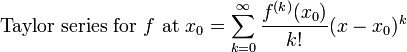$\mbox{Taylor series for } f \mbox{ at } x_0 = \sum_{k=0}^\infty \frac{f^{(k)}(x_0)}{k!}(x - x_0)^k$$\mbox{Taylor series for } g \mbox{ at } x_0 = \sum_{k=0}^\infty \frac{g^{(k)}(x_0)}{k!}(x - x_0)^k$

Thus, the sum of these Taylor series is:$\sum_{k=0}^\infty \frac{f^{(k)}(x_0) + g^{(k)}(x_0)}{k!}(x - x_0)^k$

The Taylor series for$f + g$ is:$\mbox{Taylor series for } f + g \mbox{ at } x_0 = \sum_{k=0}^\infty \frac{(f + g)^{(k)}(x_0)}{k!}(x - x_0)^k$

By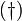$(\dagger)$, we obtain that these two Taylor series are equal coefficient-wise, hence equal.

Proof of scalar multiples

Given:$f$ is a function defined on a subset of the reals and it is infinitely differentiable at a point$x_0$ in its domain.$\lambda$ is a real number.

To prove:$\lambda f$ is infinitely differentiable at$x_0$ and the Taylor series of the function$\lambda f$ at$x_0$ is$\lambda$ times the Taylor series of$f$ at$x_0$.

Proof: By Fact (1), we have that: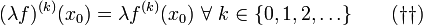$(\lambda f)^{(k)}(x_0) = \lambda f^{(k)}(x_0) \ \forall \ k \in \{0,1,2,\dots \} \qquad (\dagger\dagger)$

with equality holding whenever the right side makes sense. Since by assumption, the right side always makes sense, the left side always makes sense, so$\lambda f$ is infinitely differentiable and it makes sense to takes its Taylor series.

We note that:$\mbox{Taylor series for } f \mbox{ at } x_0 = \sum_{k=0}^\infty \frac{f^{(k)}(x_0)}{k!}(x - x_0)^k$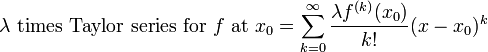$\lambda \mbox{ times Taylor series for } f \mbox{ at } x_0 = \sum_{k=0}^\infty \frac{\lambda f^{(k)}(x_0)}{k!}(x - x_0)^k$

The Taylor series for$\lambda f$ is: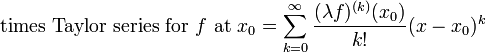$\mbox{ times Taylor series for } f \mbox{ at } x_0 = \sum_{k=0}^\infty \frac{(\lambda f)^{(k)}(x_0)}{k!}(x - x_0)^k$

By$(\dagger\dagger)$, these two Taylor series are equal coefficient-wise, hence equal.